# Difference between revisions of "Second virial coefficient"

The second virial coefficient is usually written as B or as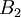$B_2$. The second virial coefficient represents the initial departure from ideal-gas behaviour. The second virial coefficient, in three dimensions, is given by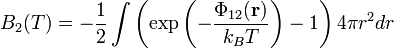$B_{2}(T)= - \frac{1}{2} \int \left( \exp\left(-\frac{\Phi_{12}({\mathbf r})}{k_BT}\right) -1 \right) 4 \pi r^2 dr$

where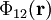$\Phi_{12}({\mathbf r})$ is the intermolecular pair potential, T is the temperature and$k_B$ is the Boltzmann constant. Notice that the expression within the parenthesis of the integral is the Mayer f-function.

In practice the integral is often very hard to integrate analytically for anything other than, say, the hard sphere model, thus one numerically evaluates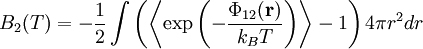$B_{2}(T)= - \frac{1}{2} \int \left( \left\langle \exp\left(-\frac{\Phi_{12}({\mathbf r})}{k_BT}\right)\right\rangle -1 \right) 4 \pi r^2 dr$

calculating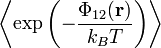$\left\langle \exp\left(-\frac{\Phi_{12}({\mathbf r})}{k_BT}\right)\right\rangle$

for each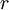$r$ using the numerical integration scheme proposed by Harold Conroy .

The Isihara-Hadwiger formula was discovered simultaneously and independently by Isihara    and the Swiss mathematician Hadwiger in 1950    The second virial coefficient for any hard convex body is given by the exact relation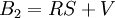$B_2=RS+V$

or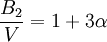$\frac{B_2}{V}=1+3 \alpha$

where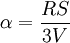$\alpha = \frac{RS}{3V}$

where$V$ is the volume,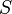$S$, the surface area, and$R$ the mean radius of curvature.

## Hard spheres

For the hard sphere model one has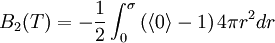$B_{2}(T)= - \frac{1}{2} \int_0^\sigma \left(\langle 0\rangle -1 \right) 4 \pi r^2 dr$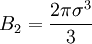$B_{2}= \frac{2\pi\sigma^3}{3}$

Note that$B_{2}$ for the hard sphere is independent of temperature. See also: Hard sphere: virial coefficients.

## Van der Waals equation of state

For the Van der Waals equation of state one has: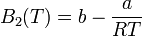$B_{2}(T)= b -\frac{a}{RT}$

## Excluded volume

The second virial coefficient can be computed from the expression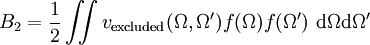$B_{2}= \frac{1}{2} \iint v_{\mathrm {excluded}} (\Omega,\Omega') f(\Omega) f(\Omega')~ {\mathrm d}\Omega {\mathrm d}\Omega'$

where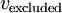$v_{\mathrm {excluded}}$ is the excluded volume.

## Admur and Mason mixing rule

The second virial coefficient for a mixture of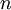$n$ components is given by (Eq. 11 in )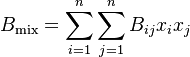$B_{ {\mathrm {mix}} } = \sum_{i=1}^{n} \sum_{j=1}^{n} B_{ij} x_i x_j$

where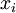$x_i$ and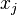$x_j$ are the mole fractions of the$i$th and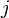$j$th component gasses of the mixture.

## Unknown

()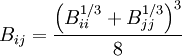$B_{ij} = \frac{\left(B_{ii}^{1/3}+B_{jj}^{1/3}\right)^3}{8}$